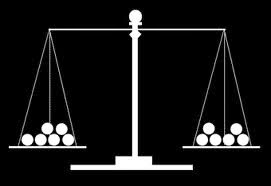# Web Puzzles

Showing posts with label stimulate brain activity. Show all posts

## 12 BallsYou have twelve balls that all weigh the same except one, which is either slightly lighter or slightly heavier. The only tool you have is a balance scale that can only tell you which side is heavier.

Using only three weighings, how can you deduce, without a shadow of a doubt, which is the odd one out, and if it is heavier or lighter than the others?

### 12 Balls Puzzle Solution

We found a good solution to this puzzle on the Cut The Knot website. They show a solution taken from the book Mathematical Spectrum, by Brian D. Bundy. Bundy shows two solutions: the first one requires different courses of action depending on the outcome of previous weighings, so it is not particularly elegant or easy to remember. The second solution involves a fixed course of action in all circumstances, and is the one that follows.

In this method we weigh four specified balls against four other specified balls in each of the three weighings and note the result. If we observe say the left hand side of the balance, then for an individual weighing there are three possible alternatives: the left hand side is heavy (>), light (<) or equal (=) as compared with the right hand side of the balance.

Since three weighings are allowed, the number of different results that can be obtained is just the number of arrangements (with repetitions allowed) of the three symbols >, <, =, i.e. 3 3 = 27. If we use all twelve balls in the three weighings, and ensure that no particular ball appears on the same side of the balance in all three weighings, the outcomes >>>, <<<, === are not possible. We thus have only 24 possible outcomes and we shall show that it is possible to set up a one to one correspondence between these 24 outcomes and the conclusion that a particular ball among the twelve is heavy or light.

The 24 outcomes can be divided into two groups of twelve in each group. If we call the reverse of an outcome the outcome obtained by replacing > by <, < by > and leaving = unchanged, one group of twelve will be the reverses of the other group and vice versa. We can thus write the 24 outcomes in the form of two arrays, each array having three rows (the three weighings) and twelve columns (the twelve balls), so that each row contains four >'s, four <'s and four ='s. Thus we have, for example,
> > > >   < < < <   = = = =
< = = =   < < > >   > < > =
=
> < =   > = < >   > = < <
A
B C D   E F G H   I J K L
<
< < <   > > > >   = = = =
> = = =   > > < <   < > < =
= < > =   < = > <   < = > >

We consider just the top array, and for each weighing (row) place the balls corresponding to a > in the left, and the balls corresponding to a < in the right of the balance. Thus we would weigh A, B, C, D against E, F, G, H; then G, H, I, K against A, E, F, J; and finally B, E, H, I against C, G, K, L. The results of these three weighings as observed on the left of the balance are noted. If the outcome is >, <, = we conclude that ball A is heavy; if >, =, > ball B is heavy, etc; if =, =, < ball L is heavy. If we obtain an outcome that appears in the lower array, we conclude that the corresponding ball is light. Thus for <, >, = ball A is light, etc; =, =, > means ball L is light.